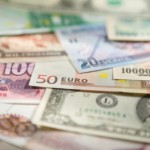Currency Converter

Exchange Rate Calculator

Currency Converter – About

Currency Converter helps you convert a value to a wide variety of currencies, this tool can also calculate the comparison between these currencies and the gold price.

Currency Converter – FormulaExchange rate: R = \$1.85 / €1.00 = 1.85 \$/€

Amount of dollars to
convert = D

Number of euros desired = E

Formula:

E = D / R

or

D = E x R

…and this is the basic formula for currency conversion used in currency converter.